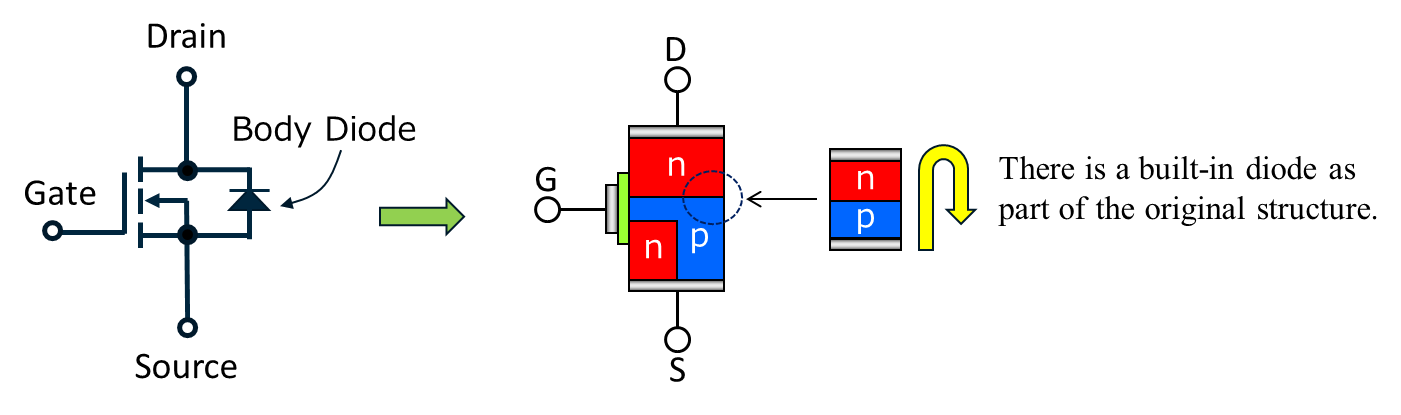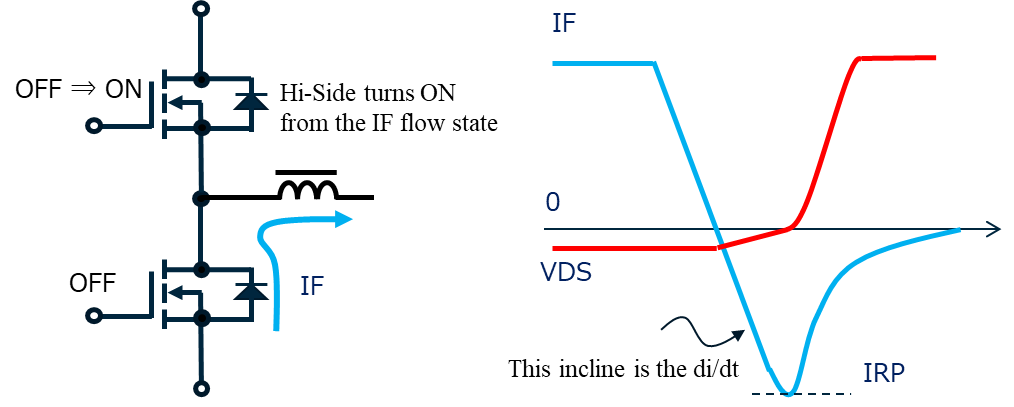# MOSFET di/dt capability

### MOSFET di/dt refers to the

1. current rate of change
2. of the recovery current
3. which flows to the MOSFET body diode.

If di/dt, which is the rate of change of the recovery current which flows to the body diode, is high, there is a risk the MOSFET could be damaged. The di/dt capability is how much di/dt the MOSFET can withstand.

•This diode can pass current even when the MOSFET is in the OFF state.
The diode is a PN diode, so it has a high reverse recovery time, and generates a high recovery current.

If the di/dt and IRP are high, there is a risk the MOSFET could be damaged. If the IRP is the rated current, the guaranteeable di/dt value is sometimes noted in the MOSFET catalog.
There are frequent problems with the characteristics of these diodes in inverters, so recently higher speed body diode products are also being developed.

•